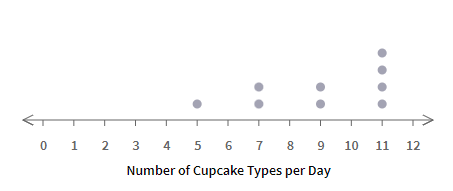ResourcesTestimonials
Plans
ResourcesTestimonials
Plans
Worksheet

# Find Mean, Median, Mode, Range From Dot Plots Worksheet

## 8 problems"Find Mean, Median, Mode, Range from Dot Plots" is a helpful tool for 6th grade mathematics students to understand and apply statistical concepts. In this worksheet, students are presented with a dot plot, a visual representation of data that displays the frequency of data points. This worksheet includes questions that require students to find the mean, median, mode, and range of the data set represented by the dot plot.

Statistics
6.SP.B.5.C

For example: The dot plot shows the number of different types of cupcakes available on different days.Find the mean and median for the number of types of cupcakes available per day.

Solution:

Data set represented by the dot plot: 5, 7, 7, 9, 9, 11, 11, 11, 11

Mean = Sum of all data point/number of data points

=  (5+7+7+9+9+11+11+11+11)/9

= 81/9

= 9

Number of data points is 9. The middle value of 5, 7, 7, 9, 9, 11, 11, 11, 11 data set is 9.

Therefore, the mean is 9 and the median is 9.

For example: The dot plot shows the number of different types of cupcakes available on different days.Find the mean and median for the number of types of cupcakes available per day.

Solution:

Data set represented by the dot plot: 5, 7, 7, 9, 9, 11, 11, 11, 11

Mean = Sum of all data point/number of data poin...

Show all

### What teachers are saying about BytelearnWhat teachers are sayingStephen Abate
19-year math teacher
Carmel, CA
Any math teacher that I know would love to have access to ByteLearn.Jennifer Maschino
4-year math teacher
Summerville, SC
“I love that ByteLearn helps reduce a teacher’s workload and engages students through an interactive digital interface.”Rodolpho Loureiro
Dean, math program manager, principal
Miami, FL
“ByteLearn provides instant, customized feedback for students—a game-changer to the educational landscape.”